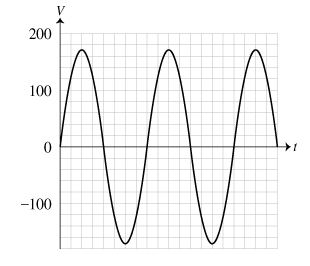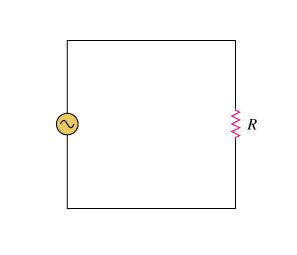# Problem: What is the rms value Vrms of the voltage plotted in the graph?Part BWhen a lamp is connected to a wall plug, the resulting circuit can be represented by a simplified AC circuit, as shown in the figure. Here the lamp has been replaced by a resistor with an equivalent resistance R = 120 Ω. What is the rmsvalue Irms of the current flowing through the circuit?What is the average power Pavg dissipated in the resistor?

###### FREE Expert Solution

Part A

Root mean square voltage,

$\overline{){{\mathbf{V}}}_{\mathbf{r}\mathbf{m}\mathbf{s}}{\mathbf{=}}\frac{{\mathbf{V}}_{\mathbf{p}\mathbf{e}\mathbf{a}\mathbf{k}}}{\sqrt{\mathbf{2}}}}$

$\overline{){{\mathbf{I}}}_{\mathbf{r}\mathbf{m}\mathbf{s}}{\mathbf{=}}\frac{{\mathbf{V}}_{\mathbf{r}\mathbf{m}\mathbf{s}}}{\mathbf{R}}}$

$\overline{){{\mathbf{P}}}_{{\mathbf{avg}}}{\mathbf{=}}{{\mathbf{V}}}_{\mathbf{r}\mathbf{m}\mathbf{s}}{{\mathbf{I}}}_{\mathbf{r}\mathbf{m}\mathbf{s}}}$

Vpeak = 170 V

91% (53 ratings)###### Problem DetailsWhat is the rms value Vrms of the voltage plotted in the graph?

Part BWhen a lamp is connected to a wall plug, the resulting circuit can be represented by a simplified AC circuit, as shown in the figure. Here the lamp has been replaced by a resistor with an equivalent resistance R = 120 Ω. What is the rmsvalue Irms of the current flowing through the circuit?

What is the average power Pavg dissipated in the resistor?